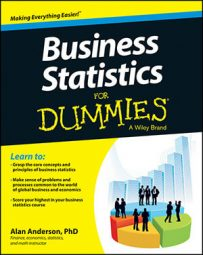When you're working with populations and samples (a subset of a population) in business statistics, you can use three common types of measures to describe the data set: central tendency, dispersion, and association.

By convention, the statistical formulas used to describe population measures contain Greek letters, while the formulas used to describe sample measures contain Latin letters.

## Measures of central tendency

In statistics, the mean, median, and mode are known as measures of central tendency; they are used to identify the center of a data set:

• Mean: The value between the largest and smallest values of a data set, obtained by a prescribed method.

• Median: The value which divides a data set into two equal halves

• Mode: The most commonly observed value in a data set

Samples are randomly chosen from populations. If this process is carried out correctly, each sample should accurately reflect the characteristics of the population. So, a sample measure, such as the mean, should be a good estimate of the corresponding population measure. Consider the following examples of mean:

Population mean:

This formula simply tells you to add up all the elements in the population and divide by the size of the population.

Sample mean: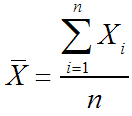The process for computing this is exactly the same; you add up all the elements in the sample and divide by the size of the sample.

In addition to measures of central tendency, two other key types of measures are measures of dispersion (spread) and measures of association.

## Measures of dispersion

Measures of dispersion include variance/standard deviation and percentiles/quartiles/interquartile range. The variance and standard deviation are closely related to each other; the standard deviation always equals the square root of the variance.

The formulas for the population and sample variance are:

Population variance: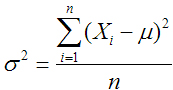Sample variance: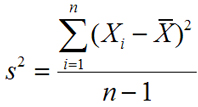Percentiles split up a data set into 100 equal parts each consisting of 1 percent of the values in the data set. Quartiles are a special type of percentiles; they split up the data into four equal parts. The interquartile range represents the middle 50 percent of the data; it's calculated as the third quartile minus the first quartile.

## Measures of association

Another type of measure, known as a measure of association, refers to the relationship between two samples or two populations. Two examples of this are the covariance and the correlation:

Population covariance: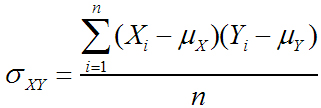Sample covariance: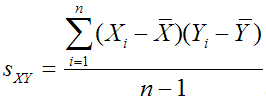Population correlation: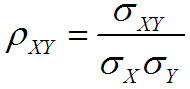Sample correlation: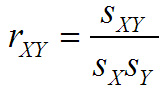The correlation is closely related to the covariance; it's defined to ensure that its value is always between negative one and positive one.